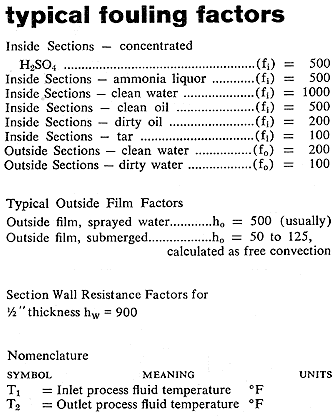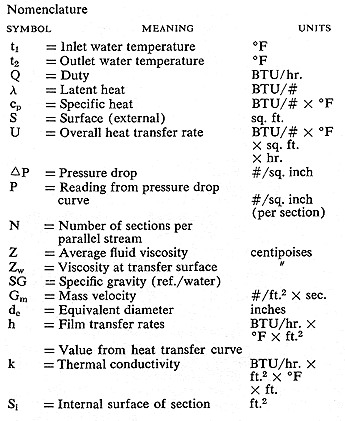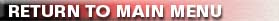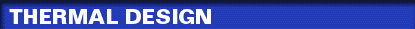Rating a cooler or condenser utilizing AX Sections follows the method generally accepted for other types of heat transfer equipment, since the same physical and thermodynamic laws apply. Knowing inlet and outlet process temperature, quantity of process fluid, its heat content (either specific or latent, or both), and quantity and summer temperature of the available cooling water, the duty is calculated in BTU/hr: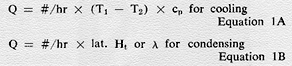The water temperature rise and final water temperature are then obtained as follows: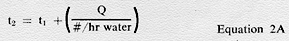With all terminal temperatures known, the logarithmic mean temperature difference is found by determining the terminal differences (noting whether flows are countercurrent or concurrent) and applying the formula: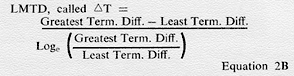A trial overall heat transfer rate Ut is selected and the basic heat transfer equation is solved for a tentative figure for surface.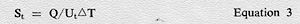From this tentative value of S, the number of Sections of the desired type can be obtained from values for external surface. With this number of Sections a trial arrangement is made, taking into consideration various limiting factors of dimension and flow quantity. The pressure drop of this trial unit can then be determined from the appropriate curve, properly corrected for the physical characteristics of the fluid being cooled. In turbulent flow the pressure drop varies as the .26 power of the viscosity, as the 1.77 power of the velocity and inversely as the density. A correction for tube wall viscosity is also applicable. Thus: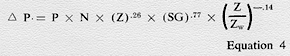Having determined the pressure drop, and made whatever adjustments in arrangements are necessary to bring the figures in line with the specified requirements, the actual available heat transfer rate can be calculated from the curve, which represents the basic heat transfer relationship, First a value for mass velocity is determined: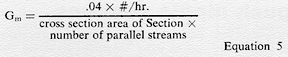then a figure N, the Reynolds number is calculable in convenient units.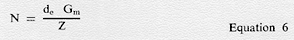Using this value of N, a reading from the curve is employed in the following formula to give a film rate "hi" for the fluid flowing inside the sections.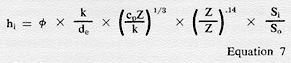This value of hi is then combined with inside and outside fouling factors, the resistance of the Section wall, and the outside film transfer rate, from values given in the accompanying table.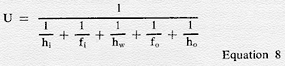The fouling factors are generally used where no abnormal operating conditions exist, and are not to be considered inflexible. After applying the value of U obtained from Equation 8 to the basic formula in Equation 3 the validity of the original trial rate is confirmed or disproved. The arrangement, type and number of Sections can be adjusted to make the most economical unit and the values of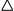P and U rechecked. In determining the number of stacks in a spray installation (not fully evaporative as described below) the quantity of cooling water per stack must be considered. For Type AX Sections, this should be held between 12 and 42 GPM. Maximum stack height should not exceed 33 Sections except for unusual conditions, although 20 is normal maximum for acid coolers. If design conditions dictate more than one stack in series per parallel stream, a correction must be made in the log mean temperature difference (see Equation 2B). If the flow in each stack is countercurrent with the water, the following method is used to find the Correct LMTD: Water quantity over each stack may be safely assumed to be equal. The overall U should be nearly constant with no change of state, and equal surface must be designed into each stack. For other conditions, the zoning can be worked out most simply by a cut and try procedure. The temperature of the hot fluid leaving the first (hottest) series pass (Tx) is found as follows: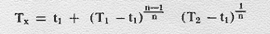where n is the number of stacks in series. The temperature of the water leaving this stack tx is found by determining the duty performed in this first pass in the manner explained by Equations 1A and 2A. It may be that a maximum water temperature in the system will be established as a limit, this could affect the design at this point. Knowing values of T1, TX, t1, and TX, all temperatures for the first pass, use Equation 2B to determine the LMTD, for this pass. Then the Corrected LMTD can be found as follows: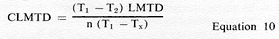EVAPORATIVE COOLING CALCULATIONS In developing the water temperature conditions for evaporative operation, from which a log mean temperature difference can be developed, the controlling factors are ambient wet bulb temperature and the desired outlet temperature of the fluid to be cooled. The arithmetic difference between these temperatures is called gross temperature difference (GTD). One third of the GTD can be safely considered as the approach between minimum water temperature (t1 in LMTD calculations), but this approach should not be taken as less than 10 degrees F, so that t1 = twb + 10 degrees F minimum (maximum is generally 20 degrees F). The range of temperature for the recirculated water can be taken as one third of GTD, so that the total quantity of water required can be determined by the following equation. (The maximum temperature of the total quantity of water is designated tr):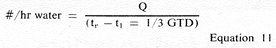All of the recirculated water does not come in contact with the Sections. The general layout permits approximately 60% of the water to fall freely. This freefall performs a major part of the cooling function due to air contact. Maximum temperature (t2) of the 40% in direct contact with the heat transfer surfaces is therefore considerably higher than tr. Value of this temperature difference between water in contact and falling freely will be: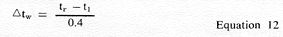and the maximum temperature of this water for purposes of calculating the log MTD will be: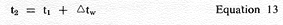From the values of t1 and t2, with known values T1 and T2 of the fluid to be cooled, the log mean temperature difference can be determined. The makeup water in #/hr required for the installation can be calculated as the duty in BTU/hr divided by the latent heat of water, which is approximately 1000 BTU/#. This figure gives the actual evaporation loss, and to this must be added an allowance for blowdown and drift. This amount is usually equal to the evaporation loss. Therefore twice the calculated amount of water evaporated will serve as a safe make-up requirement.